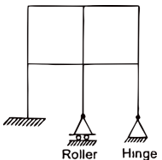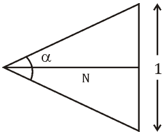Engineering Jobs   »   Civil Engineering Quiz

# DFCCIL’21 CE: Weekly Practices Quiz. 22-Aug-2021

Quiz: Civil Engineering
Exam: DFCCIL
Topic: Miscellaneous

Each question carries 1 mark
Negative marking: 1/4 mark
Time: 12 Minutes

Q1. Irrigation water having SAR value of 20, is called
(a) Very high sodium water
(b) High sodium water
(c) Medium sodium water
(d) Low sodium water

Q2. Specific gravity of sand deposit is 2.50 having the void ratio 0.65 the critical hydraulic gradient for the sand deposit:
(a) 0.85
(b) 0.90
(c) 0.75
(d) 1.0

Q3. The major constituent in Argillaceous rocks
(a) Clay
(b) Lime
(c) Silica
(d) Granite powder

Q4. If α is the angle of crossing, and then the number of crossing ‘N’ according to centre line method is given by:
(a) 1/2 cot α/2
(b) cot α/2
(c) cot⁡α
(d) 1/2 cosec α/2

Q5. According to the limit state method, neutral axis is said to be critical when:
(a) All of the above
(b) Strain in steel reaches its ultimate value earlier than strain in concrete
(c) Strain in steel and strain in concrete reach their ultimate value simultaneously
(d) Strain in concrete reaches its ultimate value earlier than strain in steel

Q6. A water supply scheme has to be designed for a city having a population of 100,000 people. If the average consumption is 250 Lpcd the estimated average daily draft is –
(a) 35 MLD
(b) 25 MLD
(c) 45 MLD
(d) 15 MLD

Q7. If the bulk modulus is K, modulus of Elasticity is E and Poisson’s ratio is 1/m, then which of the following is true?
(a) E = 3K (1+2/m)
(b) E = 3K (1-1/m)
(c) E = 3K (1-2/m)
(d) E = 3K (1+1/m)

Q8. If the fore bearing of the line AB is 119°48’ then back baring is:
(a) 299° 48’
(b) 209°48’
(c) 119°48’
(d) 319°48’

Q9. If the mass density of a fluid is 789 kg/m³, Taking g = 9.806 m/sec². Specific volume will be
(a) 0.126 m³/kN
(b) 0.122 m³/kN
(c) 0.128 m³/kN
(d) 0.132 m³/kN

Q10. The maximum bending moment due to an isolated load in a three-hinged parabolic arch (span, I, rise h) having one of its hinges at the crown, occurs on either side of the crown at a distance
(a) l/4
(b) h/4
(c) l/((2√3) )
(d) l/((3√2) )

Q11. What is the kinematic indeterminacy for the frame shown above? (Member inextensible)(a) 6
(b) 11
(c) 12
(d) 21

Q12. The Sequence of four stages of survey in a highway alignment is
(a) reconnaissance, map study, preliminary survey and detailed survey
(b) map study, preliminary survey, reconnaissance and detailed survey
(c) map study, reconnaissance, preliminary survey and detailed survey
(d) preliminary survey, map study reconnaissance and detailed survey

Solutions

S1. Ans.(b)
Sol.

 S. No. Sodium Absorption rate (SAR) value Classification 1. 0-10 Low sodium water 2. 10-18 Medium sodium water 3. 18-26 High sodium water 4. >26 Very high sodium water

S2. Ans.(b)
Sol. G=2.50
e=0.65
=0.909

S3. Ans.(a)
Sol. Argillaceous rock → In these rocks, the major constituent is clay. These are brittle in nature and can’t withstand shock.
Ex. Laterite, slates, kaoline.
Siliceous rocks → In these rocks, silica is predominates. They are hard and durable.
Ex. Granite, sandstone. Quartzite.

S4. Ans.(a)
Sol.If crossing angle α, Then No. of crossing N is given by centre line method-
Tan (α/2)=(1/2)/N
N = 1/(2Tan (α/2) )
▭(N=1/2 cot⁡(α/2) )

S5. Ans.(c)
Sol. When strain is steel & strain in concrete reach their ultimate value simultaneously then Neutral axis is said to be critical (Balanced section).

S6. Ans.(b)
Sol. Given, Population = 100,000
Average consumption = 250 lpcd
Average daily draft = 100,000×250
= 25×10^6 l.
= 25 MLD

S7. Ans.(c)
Sol. The relation in the modulus of elasticity, bulk modules and Poisson’s Ratio is
E = 3 K (1-2 μ) Here μ = 1/m
∴ E = 3K (1 – 2/m)

S8. Ans.(a)
Sol. Fore bearing of line ‘AB’ = 119°48’
Fore baring is less than 180° so we calculate back bearing by adding 180°. If fore bearing greater than 180° than we subtract 180° from it.
Back bearing of line AB = 180°+〖119°48〗^’=〖299°48〗^’

S9. Ans. (c)
Sol. Specific volume – It is defined as the number of cubic meters Occupied by one kilogram of a particular substance.
Weight density (γ) = (mass )/(volume )
ρg = 1/V
V = 1/ρg
V = 1/(789 ×9.806 )
V = 0.1277

V≈ 0.128 m³/kN

S10. Ans.(c)
Sol. The maximum bending moment due to an isolated load in a three-hinged parabolic arch (span l, rise h) having one of its hinges at the crown, occurs on either side of the crown at a distance of l/(2√3).

S11. Ans.(b)
Sol. No.of inextensible members (m) =10
No.of joints (j) =9
No.of external reactions (r_e) =6
Internal hinged reactions (r_r) =0
Kinematic indeterminacy (D_k) =3j-r_e-m+r_r
=(3×9)-6-10+0
▭(D_k=11)

S12. Ans.(c)
Sol. The sequence of four stages of survey in a highway alignment is
Map study → Reconnaissance → preliminary Survey → Detailed Survey

Sharing is caring!

Thank You, Your details have been submitted we will get back to you.